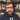Configuring Complex Luigi Pipelines

Better Ways of Configuring Complex Luigi Pipelinesby Patrick Smith

Luigi is a great tool for orchestrating complex data analysis pipelines. One potential pain point, however, is how to configure a pipeline with many tasks and, potentially, many parameters. We present a method of configuring the tasks that hopefully makes life simpler.

Consider a simple example pipeline:

import luigi

foo = luigi.Parameter()

def output(self):

def run(self):
with self.output().open('w') as f:
f.write('Foo is {}'.format(self.foo))

foo = luigi.Parameter()
bar = luigi.Parameter()

def requires(self):

def output(self):

def run(self):
with self.output().open('w') as f:
f.write('Bar is {}'.format(self.bar))

foo = luigi.Parameter()
bar = luigi.Parameter()

def requires(self):

def output(self):

def run(self):
with self.output().open('w') as f:
f.write('Bar is {}'.format(self.bar))

foo = luigi.Parameter()
bar = luigi.Parameter()
baz = luigi.Parameter()

def requires(self):
return {
}

def output(self):

def run(self):
with self.output().open('w') as f:
f.write('Baz is {}'.format(self.baz))

This defines a simple dependency graph like:

The pipeline can then be run on the command line (assuming the above code is stored in a file called luigi_example.py):

luigi --module luigi_example TaskD --foo 'foo' --bar 'bar' --baz 'baz'

One issue that you may notice is that some parameters are defined on tasks, but are just passed through to their dependencies. For example, TaskB does not use the foo parameter in its run method, but requires it in order to define the TaskA dependency in requires.

It is possible to specify the parameters only on the classes that actually use the parameters instead:

import luigi

foo = luigi.Parameter()

def output(self):

def run(self):
with self.output().open('w') as f:
f.write('I am {}'.format(self))

bar = luigi.Parameter()

def requires(self):

def output(self):

def run(self):
with self.output().open('w') as f:
f.write('I am {}'.format(self))

bar = luigi.Parameter()

def requires(self):

def output(self):

def run(self):
with self.output().open('w') as f:
f.write('I am {}'.format(self))

baz = luigi.Parameter()

def requires(self):
return {
}

def output(self):

def run(self):
with self.output().open('w') as f:
f.write('I am {}'.format(self))

However, this complicates the matter of invoking the pipeline. The command to run the pipeline is now:

Configuration Files to the Rescue

Rather than specifying the parameter values on the command line, we can instead write a configuration file and specify that Luigi use the file to configure tasks. For example, we could use the following configuration file:

foo: foo

bar: bar

bar: bar

baz: baz

Assuming this is saved in a file example.cfg, we can then run the Luigi pipeline using:

Using a config file has the added benefit of being able to commit the file to source control to preserve the parameters used for generating a particular artifact of the Luigi pipeline.

The configuration file has a couple other tricks up its sleeve. In the above example, we’re still duplicating the value of the bar parameter. Since the file is parsed using ConfigParser, we can take advantage of the DEFAULT section that each task section inherits:

[DEFAULT]
bar: bar

foo: foo

baz: baz

Note, the task must still have a section defined in the file, even though it may be empty. There’s no change to the command needed to run the pipeline with this updated file: